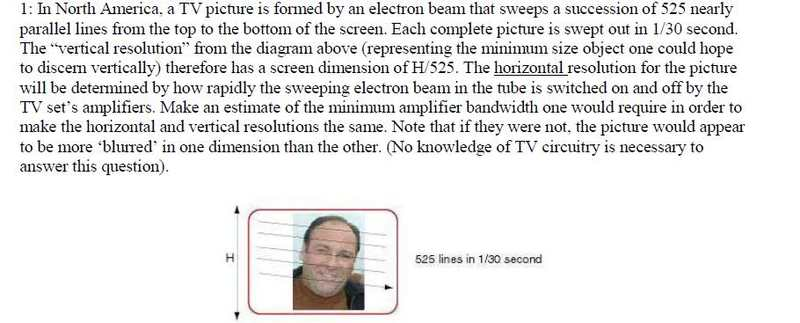# Can Someone Help Me Get Started With Amplifier Bandwidth Question

mmmboh
The question is:To tell you the truth I don't know where to start, I have read over all the teacher's notes and the chapters in the book, and I haven't seen a question similar to this at all. I am not even sure I quite get the question. Can someone help get me started please?

Thanks!

## Answers and Replies

skeptic2
(1) With 525 lines what is the maximum number of cycles that can be displayed vertically?

(2) The aspect ratio of the screen is 3:4, so number of cycles from (1) should be multiplied by what to get the number of horizontal cycles to be displayed?

(3) How long does it take the electron beam to trace one horizontal line?

(4) What would the period for one horizontal cycle be?

(5) What frequency is that equivalent to?

mmmboh
(1)T=1/30s, so maximum number of cycles = f=30 Hz I think, or would it be 525, I am not clear on the wording.
(2) I am confused on how you know the aspect ratio of the screen is 3:4, are you just assuming this is what it is is because the generic tv is 3:4?.....anyway you would have to multiply by 4/3, but I am hesitant to write this because of the 3:4 confusion.
(3)(1/30)/525
(4)(1/30)/525 wouldn't it?
(5) The inverse of number 3 or 4.

Thanks for this by the way, I was very confused by this question.

skeptic2
(1) I wasn't thinking in terms of time but just cycles. The maximum number of vertical cycles would be when the horizontal lines alternated bright and dark or a total of 212.5 cycles.

(2) Yes, the aspect ratio of the generic TV is 3:4. Back in the days when TV was invented that was considered ideal. So, if you can get 212.5 cycles vertically, in order to get square pixels you would have to multiply by 4/3 like you said.

(3) Yes

(4) What I meant was to divide the time for one horizontal scan by the number of cycles you want in that scan. [(1/30)/525] / [(4/3)*212.5].

(5) #(4) gives you the period of the cycle. To get the frequency just take the reciprocal.

mmmboh
Thanks a lot you're a big help. Just one thing, to get the bandwidth don't I have to take the difference between 2 frequencies? Right now I only have one frequency....

skeptic2
Good point. In the case of an amplifier the bandwidth is the highest frequency it amplifies minus the lowest. For a television the lowest frequency would be about 30 Hertz. So the true bandwidth would be the frequency calculated above minus 30 Hertz.

mmmboh
Thanks for the help!...seems strange that my teacher would leave out so much info.